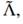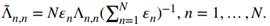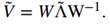# Using The Covariance Matrix V From Exercise 1 A Perform A Spectral Decomposition Vw 2664391

Using the covariance matrix V from exercise 1:

(a) Perform a spectral decomposition: VW = WΛ.

(b) Form an array 𝜀 by drawing N random numbers from a U[0, 1] distribution.

(c) Form an NxN matrixwhere(d) Compute(e) Repeat exercise 2, this time using Ṽ as covariance matrix. What allocation method has been most impacted by the re-scaling of spectral variances?

exercise 2

Using the clustered covariance matrix V from exercise 1:

(a) Compute the HRP allocations.

(b) Compute the CLA allocations.

(c) Compute the IVP allocations.

exercise 1

Given the PnL series on N investment strategies:

(a) Align them to the average frequency of their bets (e.g., weekly observations for strategies that trade on a weekly basis).

(b) Compute the covariance of their returns, V.

(c) Identify the hierarchical clusters among the N strategies.

(d) Plot the clustered correlation matrix of the N strategies.4.6/5

Price (USD)
\$